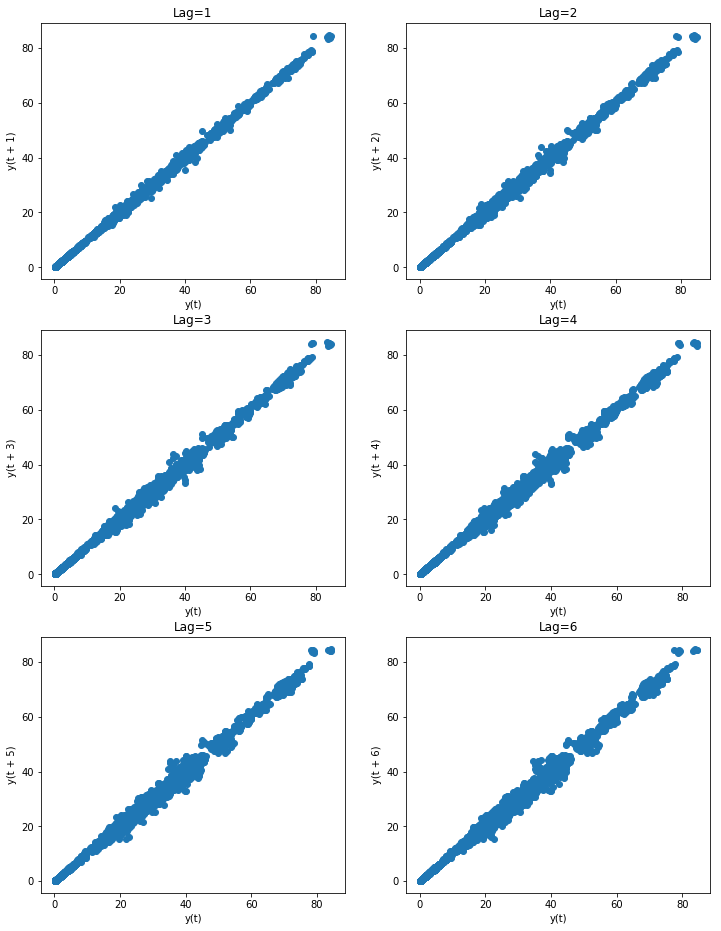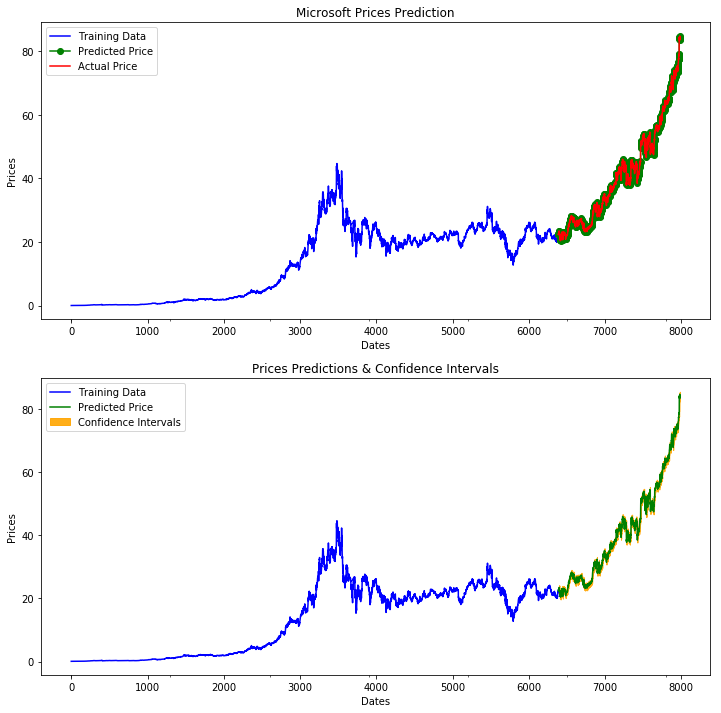# 10.1. Stock Market Prediction¶

A recent post on Towards Data Science (TDS) demonstrated the use of ARIMA models to predict stock market data with raw statsmodels. This post addresses the same problem using pmdarima’s auto-ARIMA, and ends up achieving a different result with an even lower error rate.

For this example, all we’ll need is Numpy, Pandas and pmdarima. Matplotlib is optional, but highly encouraged in order to qualitatively validate the results of the model fit. To run this example, you’ll need pmdarima version 1.5.2 or greater. If you’re running this in a notebook, make sure to include %matplotlib inline, or the plots will not show up under your cells!

Keep in mind you’ll need Python 3.6+ to install pmdarima.

import numpy as np
import pandas as pd
import matplotlib.pyplot as plt
# %matplotlib inline

import pmdarima as pm
print(f"Using pmdarima {pm.__version__}")
# Using pmdarima 1.5.2


The pmdarima module conveniently includes the dataset we’ll be using as an internal utility. Rather than carting around .csv files, you can simply load the data from the package:

from pmdarima.datasets.stocks import load_msft


 Date Open High Low Close Volume OpenInt 1986-03-13 0.06720 0.07533 0.06720 0.07533 1371330506 0 1986-03-14 0.07533 0.07533 0.07533 0.07533 409569463 0 1986-03-17 0.07533 0.07533 0.07533 0.07533 176995245 0 1986-03-18 0.07533 0.07533 0.07533 0.07533 90067008 0 1986-03-19 0.07533 0.07533 0.07533 0.07533 63655515 0

## 10.1.2. Data splitting¶

As with all statistical and ML modeling, we need to make sure we’ve split our data so we can evaluate model performance on a hold-out set. However, unlike other traditional supervised learning, time series models intrinsically introduce endogenous temporality, meaning that the values at any given point $$y_{t}$$ in our time series likely have some effect on some future value, $$y_{t+n}$$. Therefore, we cannot simply split our data randomly; we must make a clean split in our time series (and exogenous variables, if present).

As in the TDS example, we’ll use $$0.8 * dataSize$$ as our training sample.

## 10.1.3. Pre-modeling analysis¶

As you may know (if not, venture over to Tips to using auto_arima before continuing), an ARIMA model has 3 core hyper-parameters, known as “order”:

• $$p$$: The order of the auto-regressive (AR) model (i.e., the number of lag observations)
• $$d$$: The degree of differencing.
• $$q$$: The order of the moving average (MA) model. This is essentially the size of the “window” function over your time series data.

Part of the science behind the auto-arima approach is intelligently finding the proper combination of p, d, q such that you achieve the best fit. The TDS article took the approach of fixing the $$p$$ parameter at 5 after examining auto-correlations with lag plots. A lag plot can provide clues about the underlying structure of your data :

• A linear shape to the plot suggests that an autoregressive model is probably a better choice.
• An elliptical plot suggests that the data comes from a single-cycle sinusoidal model.
from pandas.plotting import lag_plot

fig, axes = plt.subplots(3, 2, figsize=(8, 12))
plt.title('MSFT Autocorrelation plot')

# The axis coordinates for the plots
ax_idcs = [
(0, 0),
(0, 1),
(1, 0),
(1, 1),
(2, 0),
(2, 1)
]

for lag, ax_coords in enumerate(ax_idcs, 1):
ax_row, ax_col = ax_coords
axis = axes[ax_row][ax_col]
lag_plot(df['Open'], lag=lag, ax=axis)
axis.set_title(f"Lag={lag}")

plt.show()As you can see, all the lags look fairly linear, so it’s a good indicator that an auto-regressive model is a good choice. But since we don’t want to allow simple visual bias to impact our decision here, we’ll allow the auto_arima to select the proper lag term for us.

### 10.1.3.1. Estimating the differencing term¶

The TDS article selected $$d=1$$ as the differencing term. But how did they make that choice? With pmdarima, we can run several differencing tests against the time series to select the best number of differences such that the time series will be stationary.

Here, we’ll use the KPSS test and ADF test, selecting the maximum value between the two to be conservative. Fortunately, in this case, both tests indicated that $$d=1$$ was the best answer, but in the case where they disagreed, we could try both or allow auto_arima to auto-select the d term.

from pmdarima.arima import ndiffs

kpss_diffs = ndiffs(y_train, alpha=0.05, test='kpss', max_d=6)

print(f"Estimated differencing term: {n_diffs}")
# Estimated differencing term: 1


Therefore, we will use $$d=1$$.

## 10.1.4. Fitting our model¶

Now it’s time to let the auto_arima method do its magic:

auto = pm.auto_arima(y_train, d=n_diffs, seasonal=False, stepwise=True,
suppress_warnings=True, error_action="ignore", max_p=6,
max_order=None, trace=True)


Notice that we preset d=n_diffs, since we’ve already settled on a value for d. However, we’re allowing our ARIMA models explore various values of p and q. After a few seconds, we arrive at the following solution:

print(auto.order)
# (0, 1, 0)


Where the TDS model was of order (5, 1, 0), we ended up selecting a significantly more simple model. But how does it perform?

## 10.1.5. Updating the model¶

Now that the heavy lifting of selecting model hyper-parameters has been performed, we can update our model by simulating days passing with our test set. For each new observation, we’ll let our model progress for several more iterations, allowing MLE to update its discovered parameters and shifting the latest observed value. Then we can measure the error on the forecasts:

from sklearn.metrics import mean_squared_error
from pmdarima.metrics import smape

model = auto  # seeded from the model we've already fit

def forecast_one_step():
fc, conf_int = model.predict(n_periods=1, return_conf_int=True)
return (
fc.tolist(),
np.asarray(conf_int).tolist())

forecasts = []
confidence_intervals = []

for new_ob in y_test:
fc, conf = forecast_one_step()
forecasts.append(fc)
confidence_intervals.append(conf)

# Updates the existing model with a small number of MLE steps
model.update(new_ob)

print(f"Mean squared error: {mean_squared_error(y_test, forecasts)}")
print(f"SMAPE: {smape(y_test, forecasts)}")
# Mean squared error: 0.34238951346274243
# SMAPE: 0.9825490519101439


In the end, our model ended up way out-performing the TDS model!

 Source MSE SMAPE pmdarima 0.342 0.983 (!!) TDS article 0.343 40.776

## 10.1.6. Viewing forecasts¶

Let’s take a look at the forecasts our model produces overlaid on the actuals (in the first plot), and the confidence intervals of the forecasts (in the second plot):

fig, axes = plt.subplots(2, 1, figsize=(12, 12))

# --------------------- Actual vs. Predicted --------------------------
axes.plot(y_train, color='blue', label='Training Data')
axes.plot(test_data.index, forecasts, color='green', marker='o',
label='Predicted Price')

axes.plot(test_data.index, y_test, color='red', label='Actual Price')
axes.set_title('Microsoft Prices Prediction')
axes.set_xlabel('Dates')
axes.set_ylabel('Prices')

axes.set_xticks(np.arange(0, 7982, 1300).tolist(), df['Date'][0:7982:1300].tolist())
axes.legend()

# ------------------ Predicted with confidence intervals ----------------
axes.plot(y_train, color='blue', label='Training Data')
axes.plot(test_data.index, forecasts, color='green',
label='Predicted Price')

axes.set_title('Prices Predictions & Confidence Intervals')
axes.set_xlabel('Dates')
axes.set_ylabel('Prices')

conf_int = np.asarray(confidence_intervals)
axes.fill_between(test_data.index,
conf_int[:, 0], conf_int[:, 1],
alpha=0.9, color='orange',
label="Confidence Intervals")

axes.set_xticks(np.arange(0, 7982, 1300).tolist(), df['Date'][0:7982:1300].tolist())
axes.legend()## 10.1.7. Conclusion¶

The TDS article provided an awesome example of how to use ARIMAs to predict stocks. Our hope in this example was to show how using pmdarima can simplify and enhance the models you build. If you’d like to run the already-setup notebook for yourself, head on over to the project’s Git page and grab the example notebook.